# Numerical Reasoning Tests: Complete Guide & Test Practice (2022)

## What is a numerical reasoning test?

A numerical reasoning test is an aptitude test measuring ability to perform calculations and interpret data in the form of charts. There are five common types of numerical reasoning tests: calculation, estimation, number sequence, word problem, and data interpretation. Most of them are in multiple-choice format. To pass the test, candidates have to make quick and accurate calculations as the test is a time constraint.

Calculation, Word problem, and Data Interpretation are the three most common question types in a numerical reasoning test. Number Sequence and Estimation are other types of questions that you may also encounter in a numerical reasoning test, but Number Sequence is often included in inductive reasoning tests and Estimation is more likely to appear in numerical reasoning tests for consulting or banking applicants.

Prevalence of each type:

Test typesPrevalence
Calculation10%
Word Problem30%
Data interpretation40%
Estimation10%
Number sequence10%

Calculation questions in numerical reasoning tests focus on assessing your math operation abilities and therefore are often given in pure calculation forms (no word context). Word problems are short context calculation problems with ENOUGH data and information for delivering the answer; data interpretation is long context problems with ABUNDANT data and information.

To improve your performance on a numerical reasoning test, you’ll need to equip yourself with the following skills:

• Make quick and accurate calculations
• Understand and create formulas in different contexts (daily, business, and finance)
• Read different types of chart presentations and filter data

As opposed to standardized math tests like GCSE or A-level exams, where you are often required to apply all kinds of mathematical techniques you’ve learned at school in impractical contexts, numerical reasoning tests are more about problem-solving in a realistic setting. These tests simulate the types of analysis you might be expected to perform in your future job.

### Aptitude Test Package

Simulating most common test publishers, this package provides you with 1400+ numerical, verbal and logical reasoning questions. Ace the aptitude test with our practical study guides tailored to each question type.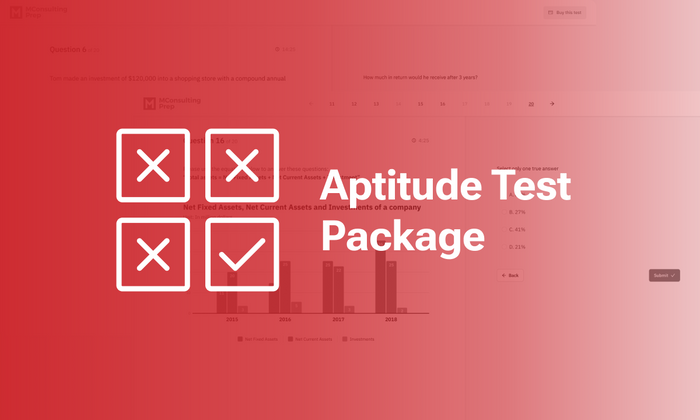## What does a numerical reasoning test examine?

Numerical reasoning tests are designed to examine the competence of test-takers in solving basic math calculations, analyzing, interpreting, and drawing conclusions from different types of data sets.

### Calculation abilities

Calculation skills are measured on numerical reasoning examinations using a variety of question formats. Numerical reasoning exams evaluate your ability to calculate by evaluating how well you do on math problems including decimals, percentages, ratios, etc., as well as fundamental arithmetic operations like addition, subtraction, multiplication, and division.

Simple math questions often don’t test your ability to think or solve problems because such questions only require quick and precise calculations.

In other types of problems, such as data interpretation, calculation abilities are not the primary assessing factor, but rather a way of assisting you in arriving at the ultimate solution.### Data analyzing competence

Numerical reasoning tests measure your ability to analyze data in questions that involve math issues in a word context or data presentations. You will be provided either adequate or abundant data to work with for the final answer.

In questions measuring data analysis skills, you must interpret and evaluate data within a given context in order to determine what information and calculations are necessary to answer the question.

### Data interpretation skill

Numerical reasoning assessments assess your data interpretation ability by asking you to derive particular inferences from your calculations. These kinds of questions are popular in numerical reasoning assessments used in consulting and business.

Questions measuring interpretation abilities frequently include workplace-based materials relevant to the function and level for which the candidate is seeking, allowing recruiters to determine whether the prospect possesses the necessary skills.

### Aptitude Test Package

Simulating most common test publishers, this package provides you with 1400+ numerical, verbal and logical reasoning questions. Ace the aptitude test with our practical study guides tailored to each question type.## Common question types

There are five types of questions that are asked in a numerical reasoning test, which are calculation, word problem, data interpretation, estimation, and number sequence.

Long and short context word problems and data interpretation are the two most common questions in numerical reasoning tests while the other three types are often adopted in gamified numerical reasoning tests.

Data interpretation questions tend to be more popular for testing high-level roles such as those in management, though they might still be questioned about basic numeracy computation and estimation.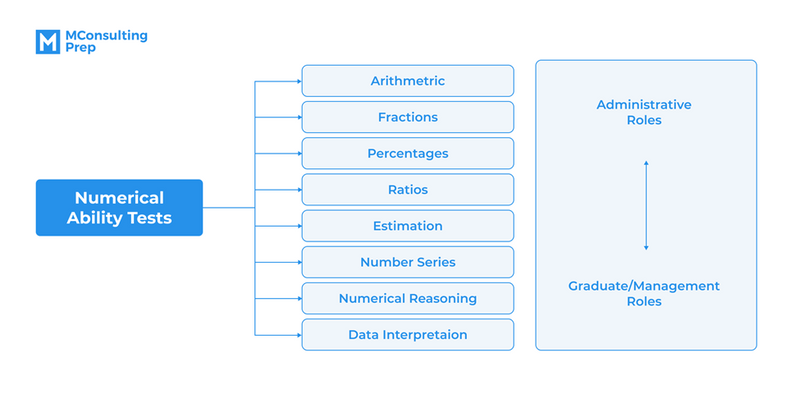Question Types in the Numerical Reasoning Test
(Source: Numerical Reasoning Psychometric Success, Paul Newton & Helen Bristoll)

### Word problem

Word problem or numerical comprehension is calculation questions with short context such as daily, business and finance context. You are provided with sufficient data instead of abundant data as distractors like data interpretation. Each question is limited to 1 minute and a calculator is allowed.

Most test suppliers provide numerical reasoning questions under this format because of two reasons. One, it is not too straightforward – candidates need to take an extra step of extracting the numbers before the actual computation. And two, math with a context is more fun and meaningful.

Numerical calculation multiple-choice questions often make use of distractors, which are options that are either very similar to the correct answer or can be achieved by making a common error.

Now, practice with these sample word problems for yourself:

1. If Millie starts school at 9:00 am and finishes at 4:15 pm, and has a total of 75 minutes of break a day, how many hours does she have school in 3 days?

2. In an employee survey of 325 employees, the response rate was six out of 10. How many employees did not complete the survey?

3. A restaurant bill is made up of the following:

Starters: \$11.75
Main courses: \$34.25
Desserts: \$14
10% service charge on total bill

How much is the bill?

4. A consultancy’s operating costs to turnover ratio is 3:20 each year. If the company’s turnover is £213,250 in Year 1, £268,460 in Year 2, and £328,915 in Year 3 what are the total operating costs for the three-year period?

5. At an exchange rate of €1.20 to the £, what is the cost (in £) of three boxes of office paper at €6.60 per box?

1. 18

2. 130

3. \$66

4. £121,594

5. £16.50

### Data interpretation

The second common question type is data interpretation questions. You may be given numerical data in the forms of tables, graphs, and charts and asked to draw inferences based on the information. Applicants for jobs requiring numerical data analysis or decision-making should be prepared to answer this type of question.

Presentation of data in this kind of question can be classified into 5 basic types:

• Tables
• Bar charts
• Line charts
• Pie charts
• Caselet (data is provided in a paragraph)

Five simple steps are required when attempting to interpret data:

Step 1: Look through the charts and understand what kind of data they present. If there are multiple charts, make sure you understand the relationship between them.

Step 2: Read the question and determine what kind of data you’ll need.

Step 3: Find the table/graph/chart that has the information you need and locate the information.

Step 4: Apply/Manipulate the information to compute the answer.

Step 5 (optional): Translate the numerical answer into a meaningful inference if the question requires you to do so.

There are typically two levels of difficulty when it comes to data interpretation questions:

• Level 1: Single chart (simple)

Questions at the intermediate level usually provide ONE table/chart/graph for you to work with and are pretty straightforward, that is, only one calculation is needed to answer each question.

• Level 2: Compound chart (advanced)

Advanced data interpretation questions often present two or more sources of information (tables/charts/graphs). They might require at least two calculations to come up with the answer, though these computations aren’t necessarily difficult. It might be tricky to locate the data you need because either there is so much information or you might need to combine charts to extract the data.

Test yourself with these two sets of data interpretation questions:

• Level 1: Single chart

The figures show a population by economic activity and district.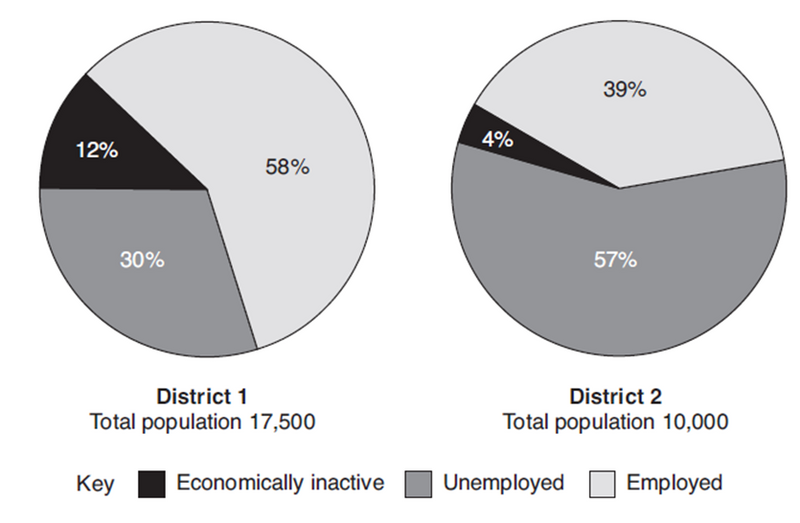1. How many people in district 1 are employed?

2. How many people across both districts are employed?

3. Express in its simplest form the ratio between economically inactive people and people not economically inactive (those unemployed or employed) in districts 1 and 2 combined.

4. What percentage of people economically inactive across both districts are residents in District 2?

1. D

2. A

3. D

4. A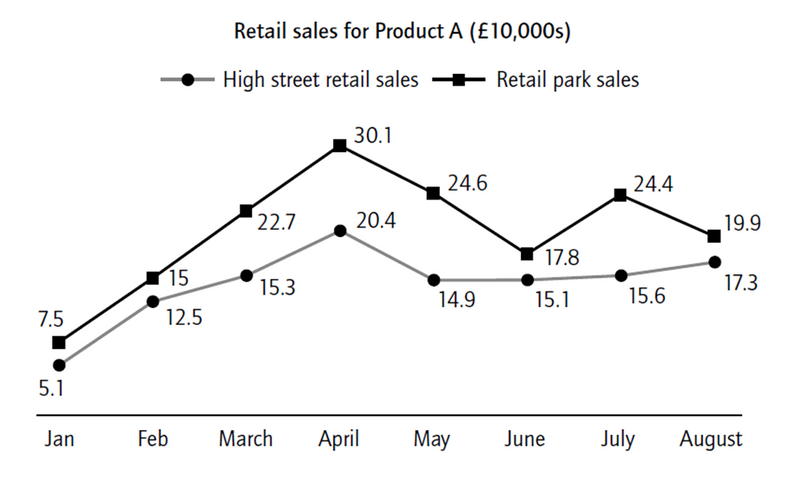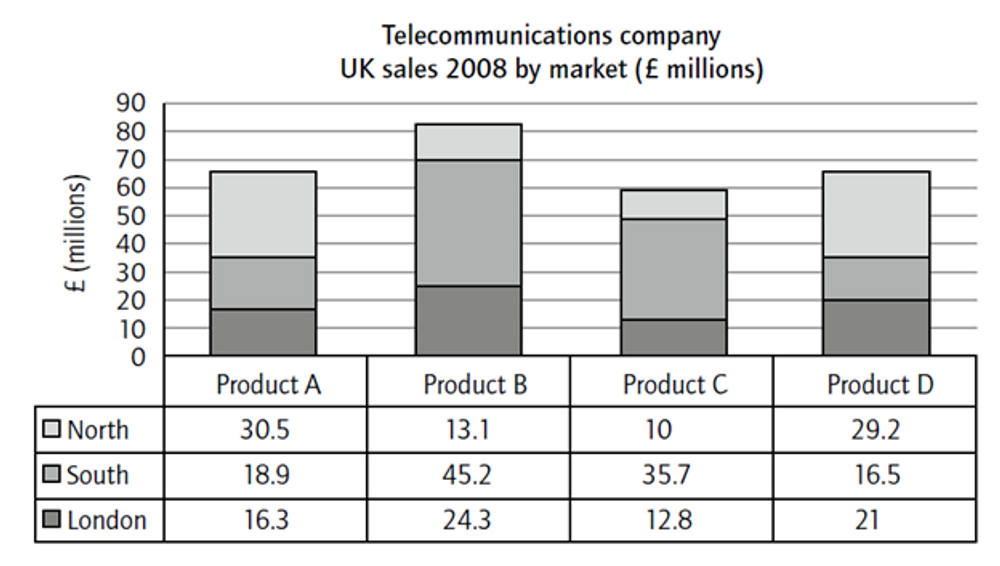1. In which two months is there the same difference between the high street and retail sales for Product A?

2. In which month was high street retail sales higher than retail park sales for Product A?

3. Between which months did both the retail park and high street sales of Product A fall?

4. In 2008 what is the difference between the UK and Asian sales (to the nearest £ million)?

5. In which two quarters were the total non-UK sales the same?

6. Due to increasing inflation, 2009’s quarterly non-UK sales are predicted to be 3%, 4%, 5%, and 6% higher than the respective 2008 quarters. What is the total sales prediction for 2009?

1. D

2. E

3. C

4. B

5. C

6. A

### Aptitude Test Package

Simulating most common test publishers, this package provides you with 1400+ numerical, verbal and logical reasoning questions. Ace the aptitude test with our practical study guides tailored to each question type.## Skills to practice for numerical reasoning tests

To handle numerical reasoning test questions, it’s necessary that you have the following skills:

• Processing math in different contexts
• Filtering data in different chart forms
• Making quick and accurate calculations/estimations

### Processing math in different contexts

In your numerical reasoning tests, you will most likely be asked to perform a lot of arithmetic calculations in various contexts. There are a great number of graphs, tables, and word problems depicting various daily, business, or finance-related contexts that you must work on for the subsequent questions. Each set of provided data might include between two and five questions, each with four or five answer choices. Your job is to determine the accurate answer based entirely on the numerical data presented.

If you want to pass your numerical reasoning test, you must learn to process arithmetic in a variety of contexts. In general, there are three primary math settings that you may encounter: daily, business, and financial.

### Filtering data in different chart forms

Filtering data in various formats is what allows you to cope with data interpretation questions (long context) when there is a lot of distracting data. The abundance of details presented in the question rather than the calculations required for the final answer choice is what makes data interpretation complex.

Since data interpretation questions are typically presented in various data forms such as tables, bars, lines, pie charts, and caselets, you can practice filtering data by attempting to solve problems in such data formats. We offer a handful of examples to help you understand how you may try to answer these questions.### Making quick and accurate calculations/estimations

Your ability to do quick and precise calculations determines whether or not you score high on the test. This ability allows you to perform simple calculations faster to save time for answering more complex math problems.

The key to becoming an expert at completing quick and correct calculations is to practice mental math and basic to advanced calculations such as big numbers, decimals, and serial calculations on a regular basis.

### Aptitude Test Package

Simulating most common test publishers, this package provides you with 1400+ numerical, verbal and logical reasoning questions. Ace the aptitude test with our practical study guides tailored to each question type.## Math formulas in numerical reasoning tests

Besides four common math operations, you’ll need a couple more math formulas when dealing with word problems and data interpretation questions. Below is the list of math formulas you need for a numerical reasoning test.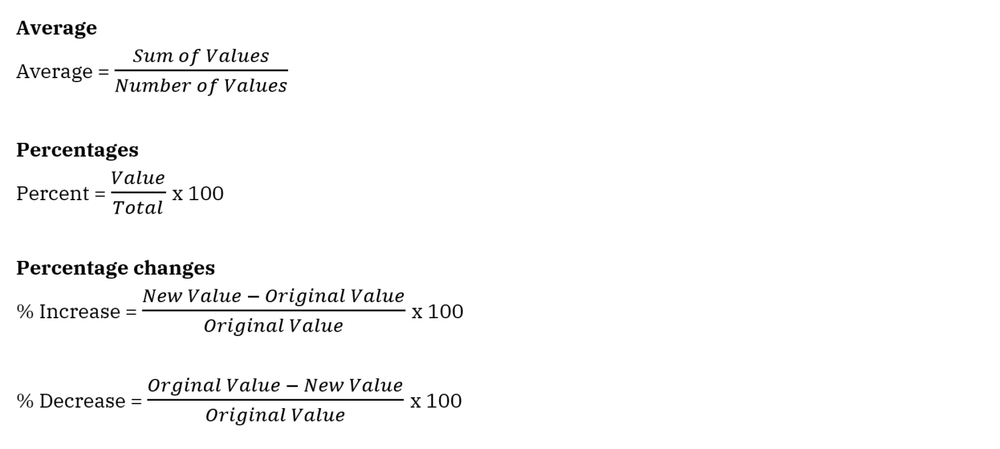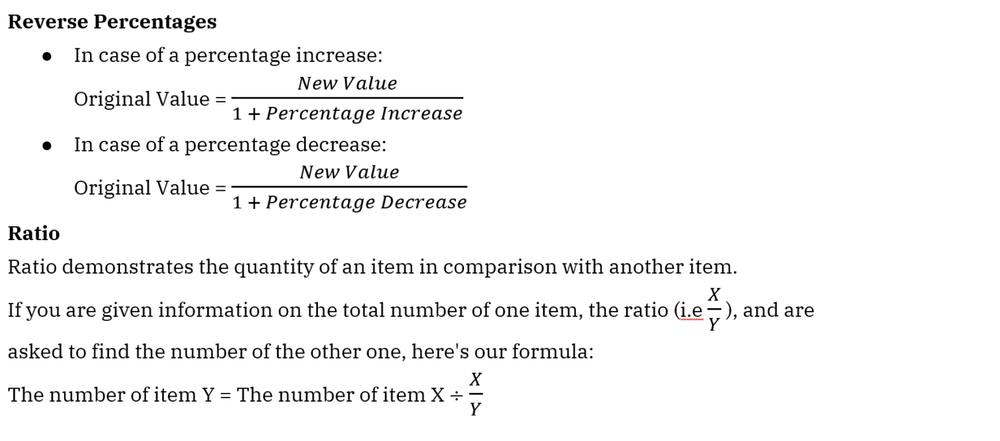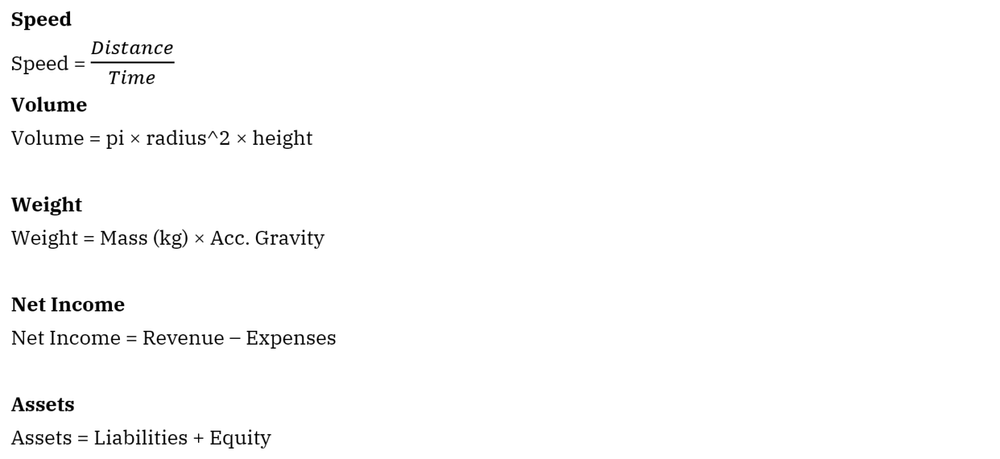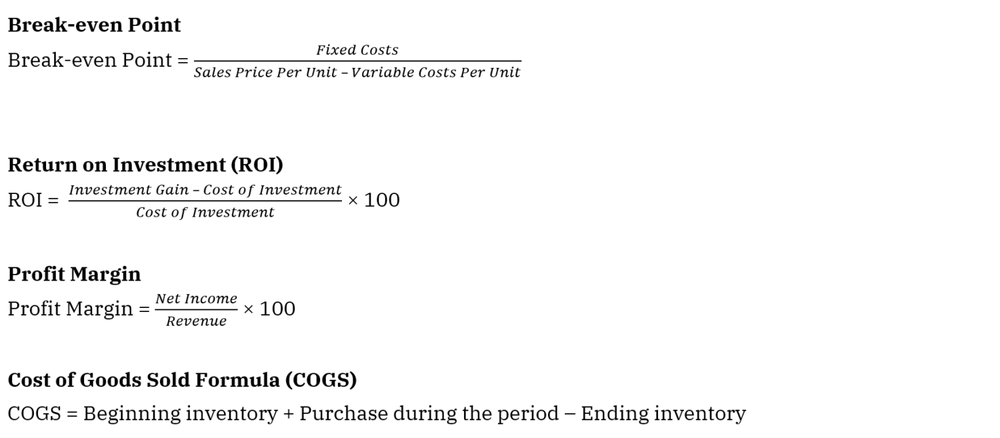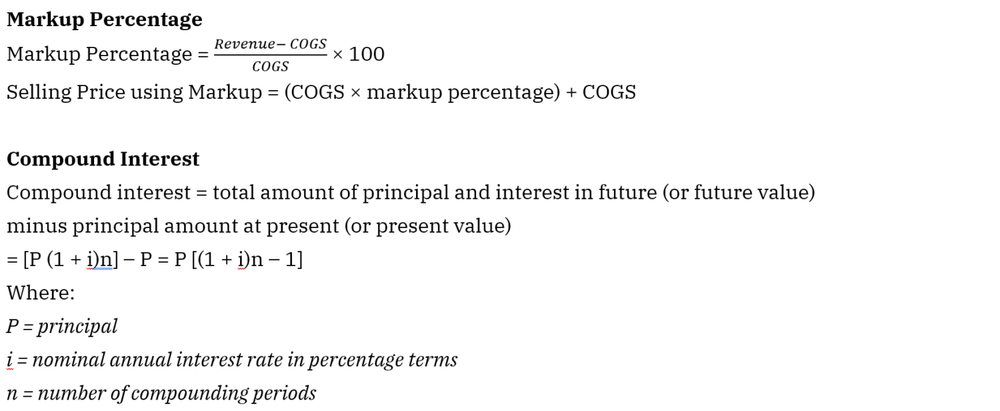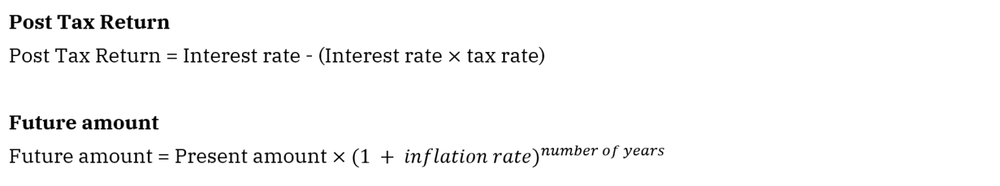### Aptitude Test Package

Simulating most common test publishers, this package provides you with 1400+ numerical, verbal and logical reasoning questions. Ace the aptitude test with our practical study guides tailored to each question type.## Popular numerical reasoning test providers

There are a number of popular numerical reasoning test providers. The list includes the following test providers:

• SHL
• Kenexa (IBM)
• TalentLens (Pearson)
• Cubiks
• Talent Q
• Sova
• Criteria Corp
• Saville
• Pymetrics

In numerical reasoning tests of such test providers, word problems and data interpretation are the two most common question types. Here’s the question prevalence breakdown: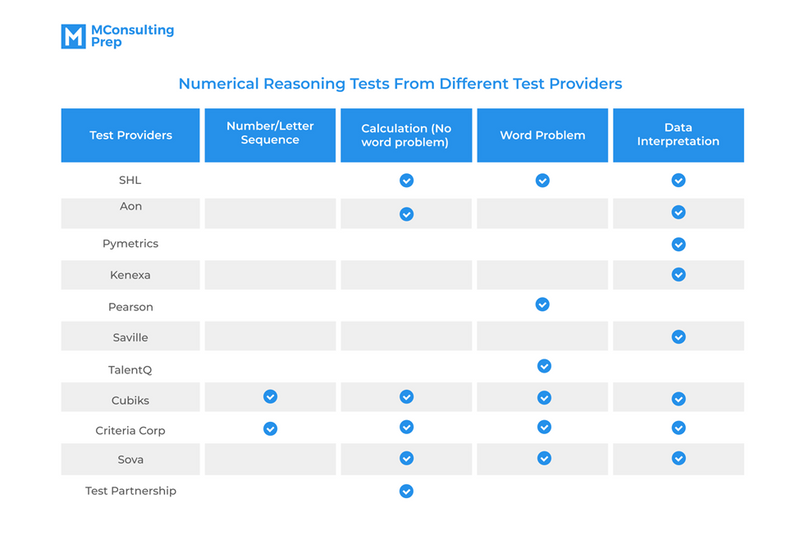Scoring in the McKinsey PSG/Digital Assessment

The scoring mechanism in the McKinsey Digital Assessment

Scoring in the McKinsey PSG/Digital Assessment

The scoring mechanism in the McKinsey Digital Assessment

Related product

## Aptitude Test Package

Simulating most common test publishers, this package provides you with 1400+ numerical, verbal and logical reasoning questions. Ace the aptitude test with our practical study guides tailored to each question type.